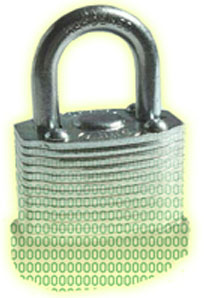# What is RSA Data EncryptionRSA

RSA, is abbrevieted from its inventors Ron Rivest, Adi Shamir, and Leonard Adleman , is a public key algorithm that is the most popular when it comes to asymmetric algorithms.RSA is a worldwide de facto standard and can be used for digital signatures, key exchange,and encryption. It was developed in 1978 at MIT and provides authenticationas well as key encryption

The security of this algorithm comes from the difficulty of factoring large numbers. The public and private keys are functions of a pair of large prime numbers, and the necessary activity required to decrypt a message from ciphertext to plaintext using a private key is comparable to factoring a product into two prime numbers.

One of the advantage of using RSA is that it can be used for encryption and digital signatures. Using its one-way function, RSA provides encryption and signature verification, and the inverse direction performs decryption and signature generation.

RSA has been used in applications; operating systems by Microsoft, Apple,Sun, and Novell; and at the hardware level in network interface cards, secure telephones, and smart cards. It can be used as a key exchange protocol, meaning it is used to encrypt the symmetric key to get it securely to its destination. RSA has been most commonly used with the symmetric algorithm DES, which is quickly being replaced with AES. So, when RSA is used as a key exchange protocol, a cryptosystem generates a symmetric key using either the DES or AES algorithm. Then the system encrypts the symmetric key with the receiver’s public key and sends it to the receiver. The symmetric key is protected because only the individual with the corresponding private key can decrypt and extract the symmetric key

Diving into Numbers

Cryptography is really all about using mathematics to scramble bits into an undecipherable form and then using the same mathematics in reverse to put the bits back into a form that can be understood by computers and people. RSA’s mathematics are based on the difficulty of factoring a large integer into its two prime factors. Put on your nerdy hat with the propeller and let’s look at how this algorithm works.

The algorithm creates a public key and a private key from a function of large prime numbers. When data are encrypted with a public key, only the corresponding private key can decrypt the data. This act of decryption is basically the same as factoring the product of two prime numbers. So, let’s say I have a secret (encrypted message), and for you to be able to uncover the secret, you have to take a specific large number and factor it and come up with the two numbers I have written down on a piece of paper. This may sound simplistic, but the number you must properly factor can be 2^300 in size. Not as easy as one may think.

The following sequence describes how the RSA algorithm comes up with the keys in the first place:

1. Choose two random large prime numbers, p and q.

2. Generate the product of these numbers: n = pq.

3. Choose a random number to be the encryption key, e. Make sure that e and (p – 1)(q – 1) are relatively prime.

4. Compute the decryption key, d. This is ed = 1 mod (p – 1)(q – 1) or d = e ^–1  mod ([p – 1][q – 1]).

5. The public key = (n, e).

6. The private key = d.

7. The original prime numbers p and q are discarded securely

We now have our public and private keys, but how do they work together?

If you need to encrypt message m with your public key (e, n), the following formula is carried out:

C = m^e mod n

Then you need to decrypt the message with your private key (d), so the following formula is carried out:

M = c^d mod n

You may be thinking, “Well, I don’t understand these formulas, but they look simple enough. Why wouldn’t someone be able to break these small formulas and be able to uncover the encryption key?” Maybe someone will one day. As the human race advances in its understanding of mathematics and as processing power increases and cryptanalysis evolves, the RSA algorithm may be broken one day. If we figure out how to quickly and more easily factor large numbers into their original prime values, all of these cards fall down and this algorithm would no longer provide the security it does today. But we have not hit that bump in the road yet, so we are all happily using RSA in our computing activities.

Article RatingWe are teams that have the same hobbies in Information Technologies and have experienced in many fields regarding Information Technologies .

### Recent Articles

Subscribe
Notify of